Courses

Top Down Parsing Notes | Study Compiler Design - Computer Science Engineering (CSE)

Computer Science Engineering (CSE): Top Down Parsing Notes | Study Compiler Design - Computer Science Engineering (CSE)

The document Top Down Parsing Notes | Study Compiler Design - Computer Science Engineering (CSE) is a part of the Computer Science Engineering (CSE) Course Compiler Design.
All you need of Computer Science Engineering (CSE) at this link: Computer Science Engineering (CSE)

TOP DOWN PARSING:
Top down parsing is the construction of a Parse tree by starting at start symbol and “guessing” each derivation until we reach a string that matches input. That is, construct tree from root to leaves.
The advantage of top down parsing in that a parser can directly be written as a program. Table-driven top-down parsers are of minor practical relevance. Since bottom-up parsers are more powerful than top-down parsers, bottom-up parsing is practically relevant.
For example, let us consider the grammar to see how top-down parser works:

S → if E then S else S | while E do S | print
E →  true | False | id

The input token string is: If id then while true do print else print.

1. Tree:
S
Input: if id then while true do print else print.
Action: Guess for S.

2.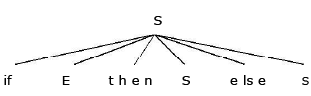3. Tree: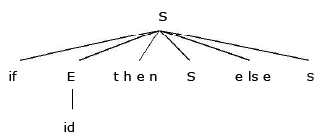Input:  id then while true do print else print.
Action: id matches; then matches; guess for S.

4. Tree: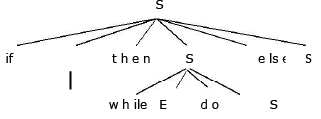Input:  while true do print else print.
Action: while matches; guess for E.

5. Tree: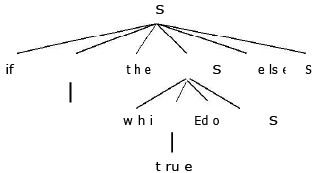Input:  true do print else print
Action:true matches; do matches; guess S.

6. Tree: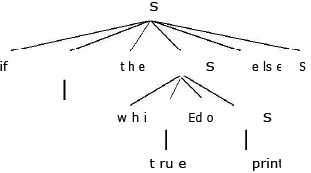Input:  print else print.
Action: print matches; else matches; guess for S.

7. Tree: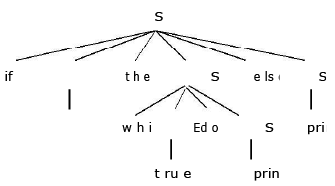Input: print.
Action: print matches; input exhausted; done.

Recursive Descent Parsing:
Top-down parsing can be viewed as an attempt to find a left most derivation for an input string. Equivalently, it can be viewd as a attempt to construct a parse tree for the input starting from the root and creating the nodes of the parse tree in preorder.

The special case of recursive –decent parsing, called predictive parsing, where no backtracking is required.
The general form of top-down parsing, called recursive descent, that may involve backtracking, that is, making repeated scans of the input.

Recursive descent or predictive parsing works only on grammars where the first terminal symbol of each sub expression provides enough information to choose which production to use.

Recursive descent parser is a top down parser involving backtracking. It makes a repeated scans of the input. Backtracking parsers are not seen frequently, as backtracking is very needed to parse programming language constructs.
Example: consider the grammar
A→ab|a
And the input string w=cad. To construct a parse tree for this string top-down, we initially create a tree consisting of a single node labeled scan input pointer points to c, the first symbol of w. we then use the first production for S to expand tree and obtain the tree of Fig(a).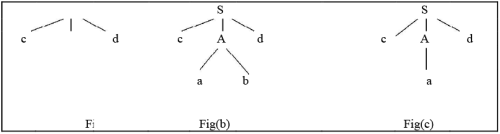The left most leaf, labeled c, matches the first symbol of w, so we now advance the input pointer to a ,the second symbol of w, and consider the next leaf, labeled A. We can then expand A using the first alternative for A to obtain the tree in Fig (b). we now have a match for the second input symbol so we advance the input pointer to d, the third, input symbol, and compare d against the next leaf, labeled b. since b does not match the d ,we report failure and go back to A to see where there is any alternative for Ac that we have not tried but that might produce a match.

In going back to A, we must reset the input pointer to position2,we now try second alternative for A to obtain the tree of Fig(c).The leaf matches second symbol of w and the leaf d matches the third symbol .

The left recursive grammar can cause a recursive- descent parser, even one with backtracking, to go into an infinite loop.That is ,when we try to expand A, we may eventually find ourselves again trying to ecpand A without Having consumed any input.

Predictive Parsing:

Predictive parsing is top-down parsing without backtracking or look a head. For many languages, make perfect guesses (avoid backtracking) by using 1-symbol look-a-head. i.e., if:
A → aI | a 2 | - - - | an.

Choose correct ai by looking at first symbol it derive. If∈is an alternative, choose it last.
This approach is also called as predictive parsing. There must be at most one production in order to avoid backtracking. If there is no such production then no parse tree exists and an error is returned.

The crucial property is that, the grammar must not be left-recursive.
Predictive parsing works well on those fragments of programming languages in which keywords occurs frequently.
For example:
stmt → if exp then stmt else stmt | while expr do stmt
| begin stmt-list end.

then the keywords if, while and begin tell, which alternative is the only one that could possibly
succeed if we are to find a statement.
The model of predictive parser is as follows: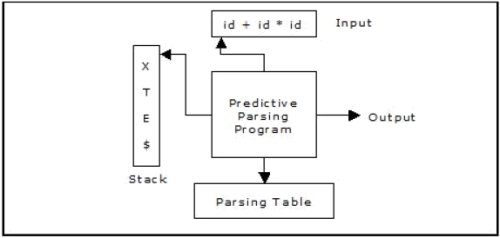A predictive parser has:

• Stack
• Input
• Parsing Table
• Output

The input buffer consists the string to be parsed, followed by \$, a symbol used as a right end marker to indicate the end of the input string.
The stack consists of a sequence of grammar symbols with \$ on the bottom, indicating the bottom of the stack. Initially the stack consists of the start symbol of the grammar on the top of \$.
Recursive descent and LL parsers are often called predictive parsers, because they operate by predicting the next step in a derivation.

The algorithm for the Predictive Parser Program is as follows: Input: A string w and a parsing table M for grammar G

Output: if w is in L(g),a leftmost derivation of w; otherwise, an error indication.

Method: Initially, the parser has \$S on the stack with S, the start symbol of G on top, and w\$ in the input buffer. The program that utilizes the predictive parsing table M to produce a parse for the input is:

Set ip to point to the first symbol of w\$; repeat
let x be the top stack symbol and a the symbol pointed to by ip; if X is a terminal or \$ then
if X = a then

pop X from the stack and advance ip else error() else
/* X is a non-terminal */

if M[X, a] = X àY1 Y2 . . . . . . . Yk then begin

pop X from the stack;
push Yk, Yk-1, . . . . . . . . . . .Y1 onto the stack, with Y1 on top; output the
production X àY1 Y2 . . . . . Yk
end
else error()
until X = \$ /*stack is empty*/

FIRST and FOLLOW: The construction of a predictive parser is aided by two functions with a grammar G. these functions, FIRST and FOLLOW, allow us to fill in the entries of a predictive parsing table for G, whenever possible. Sets of tokens yielded by the FOLLOW function can also be used as synchronizing tokens during pannic-mode error recovery.

If α is any string of grammar symbols, let FIRST (α) be the set of terminals that begin the strings derived from α. If α=>€,then € is also in FIRST(α).

Define FOLLOW (A), for nonterminals A, to be the set of terminals a that can appear immediately to the right of A in some sentential form, that is, the set of terminals a such that there exist a derivation of the form S=>αAaβ for some α and β. If A can be the rightmost symbol in some sentential form, then \$ is in FOLLOW(A).

Computation of FIRST (): To compute FIRST(X) for all grammar symbols X, apply the following rules until no more terminals or € can be added to any FIRST set. ·

• If X is terminal, then FIRST(X) is {X}.
• If X→€ is production, then add € to FIRST(X).
•  If X is nonterminal and X→Y1 Y2……Yk is a production, then place a in FIRST(X) if for some i,a is in FIRST(Yi),and € is in all of FIRST(Yi),and € is in   all of FIRST(Y1),&.. FIRST(Yi-1);that is Y1&&&. Yi-1==>¬.if ¬ is in   FIRST(Yj), for all j=,2,3&&.k, then add ¬ to FIRST(X).for example, everything in FIRST(Y1) is surely in FIRST(X).if Y1 does not derive ¬,then we add nothing more to FIRST(X),but if Y1=>¬,then we add FIRST(Y2) and so on.

FIRST (A) = FIRST (aI) U FIRST (a2) U - - - U FIRST (an) Where, A → a1 | a2 | - - - |an, are all the productions for A. FIRST (Aa) = if∈Ï FIRST (A) then FIRST (A) else (FIRST (A) - {Î}) U FIRST (a)

To compute FOLLOW (A) for all nonterminals A, apply the following rules until nothing can be added to any FOLLOW set.

• Place \$ in FOLLOW(s), where S is the start symbol and \$ is input right end marker .
• If there is a production A→αBβ,then everything in FIRST(β) except for € is placed in   FOLLOW(B).
• If there is production A→αB, or a production A→αBβ where FIRST (β) contains € (i.e.,β→€),then everything in FOLLOW(A)is in FOLLOW(B).

Example:
Construct the FIRST and FOLLOW for the grammar:

A → BC | EFGH | H
B → b
C → c | ∈
E → e |∈
F → CE
G → g
H → h | ∈

Solution: 1. Finding first () set:
1. first (H) = first (h) ∪ È first (e) = {h, e}
2. first (G) = first (g) = {g}
3. first (C) = first (c) ∪ È first (ε) = c, e}
4. first (E) = first (e) ∪ È first (e) = {e, e}
5. first (F) = first (CE) = (first (c) - {e}) È first (E) = (c, e} {e}) È {e, e} = {c, e, e}
6. first (B) = first (b)={b}
7. first (A) = first (BC) ∪ È first (EFGH) ∪ È first (H)
=first (B) È ∪ (first (E)  { e}) È ∪ first (FGH) ∪ È {h, e}
= {b, h, e} È ∪ {e} È ∪ (first (F)  {e}) ∪ È first (GH)
= {b, e, h, e} È ∪ {C, e} È ∪ first (G)
= {b, c, e, h, e} È {g} = {b, c, e, g, h, e}

2. Finding follow() sets:
1. follow(A) = {\$}
2. follow(B) = first(C) – {ε} È follow(A) = {C, \$}
3. follow(G) = first(H)  {ε} È follow(A) ={h, e}  {e} È {\$} = {h, \$}
4. follow(H) = follow(A) = {\$}
5. follow(F) = first(GH)  {ε} = {g}
6. follow(E) = first(FGH) m- {ε} È follow(F) = ((first(F)  {e}) È first(GH))  {e} È follow(F)
= {c, e} È {g} È {g}
= {c, e, g}
7. follow(C) = follow(A) È first (E)  {e} È follow (F) ={\$}
È {ε,ε} È {g} = {e, g, \$}

Example 1:
Construct a predictive parsing table for the given grammar or Check whether the given grammar is LL(1) or not.
E → E + T | T
T → T * F | F F → (E) | id

Step 1:

Suppose if the given grammar is left Recursive then convert the given grammar (and Î) into non-left Recursive grammar (as it goes to infinite loop).
E → T EI
EI → + T EI | Î TI → F TI
TI → * F TI | Î F → (E) | id

Step 2:
Find the FIRST(X) and FOLLOW(X) for all the variables.

The variables are: {E, EI, T, TI, F}
Terminals are: {+, *, (, ), id} and \$
Computation of FIRST() sets:

FIRST (F) = FIRST ((E)) U FIRST (id) = {(, id} FIRST (TI) = FIRST (*FTI) U FIRST (Î) = {*, Î}
FIRST (T) = FIRST (FTI) = FIRST (F) = {(, id} FIRST (EI) = FIRST (+TEI) U FIRST (Î) = {+, Î}
FIRST (E) = FIRST (TEI) = FIRST (T) = {(, id}

Relevant production

FOLLOW (E) = {\$} U FIRST ( ) ) = {\$, )}                                                                  F → (E)
= {+, ), \$}                                                                                                                   EI → +TEI
FOLLOW (TI) = FOLLOW (T) = {+, ), \$}                                                                   T → FTI
{*, +, ),                                                                                                 T → TI

Step 3: Construction of parsing table: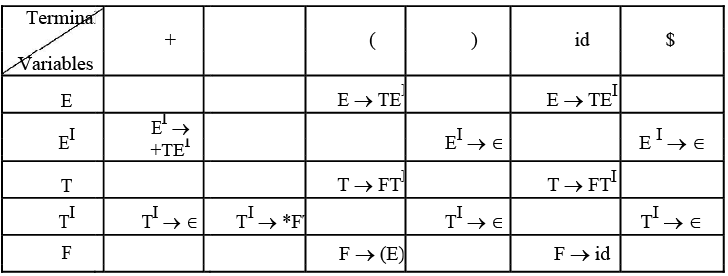Table 3.1. Parsing Table

Fill the table with the production on the basis of the FIRST( a). If the input symbol is an Î in FIRST(a), then goto FOLLOW(a) and fill a → Î, in all those input symbols.

3.1. Let us start with the non-terminal E, FIRST(E) = {(, id}. So, place the production E → TEI at ( and id.

3.2. For the non-terminal EI, FIRST (EI
) = {+, Î}. So, place the production EI → +TEI at + and also as there is a Î in FIRST(EI), see FOLLOW(EI) = {\$, )}. So write the production EI → Î at the place \$ and ). Similarly:

3.3. For the non-terminal T, FIRST(T) = {(, id}. So place the production T → FTI at ( and id.

3.4. For the non-terminal TI, FIRST (TI) = {*, Î} So place the production TI → *FTI at * and also as there is a Î in FIRST (TI), see FOLLOW (TI) = {+, \$, )}, so write the production TI → Î at +, \$ and ).

3.5. For the non-terminal F, FIRST (F) = {(, id}. So place the production F → id at id location and F → (E) at ( as it has two productions.

3.6. Finally, make all undefined entries as error. As these were no multiple entries in the table, hence the given grammar is LL(1).

Step 4: Moves made by predictive parser on the input id + id * id is: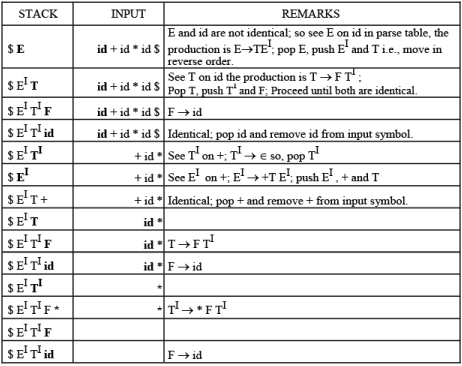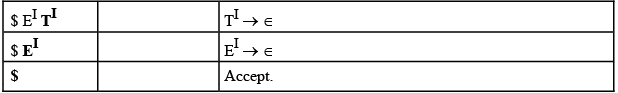Predictive parser accepts the given input string. We can notice that \$ in input and stuck, i.e., both are empty, hence accepted.

LL (1) Grammar:
The first L stands for “Left-to-right scan of input”. The second L stands for “Left-most derivation”. The ‘1’ stands for “1 token of look ahead”.
No LL (1) grammar can be ambiguous or left recursive.

If there were no multiple entries in the Recursive decent parser table, the given grammar is LL (1).

If the grammar G is ambiguous, left recursive then the recursive decent table will have atleast one multiply defined entry.

The weakness of LL(1) (Top-down, predictive) parsing is that, must predict which production to use.

Error Recovery in Predictive Parser:
Error recovery is based on the idea of skipping symbols on the input until a token in a selected set of synchronizing tokens appear. Its effectiveness depends on the choice of synchronizing set. The Usage of FOLLOW and FIRST symbols as synchronizing tokens works reasonably well when expressions are parsed.

For the constructed table., fill with synch for rest of the input symbols of FOLLOW set and then fill the rest of the columns with error term.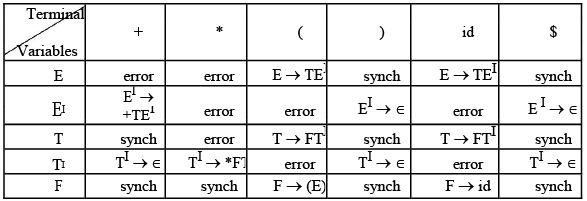If the parser looks up entry in the table as synch, then the non terminal on top of the stack is popped in an attempt to resume parsing. If the token on top of the stack does not match the input symbol, then pop the token from the stack.
The moves of a parser and error recovery on the erroneous input) id*+id is as follows: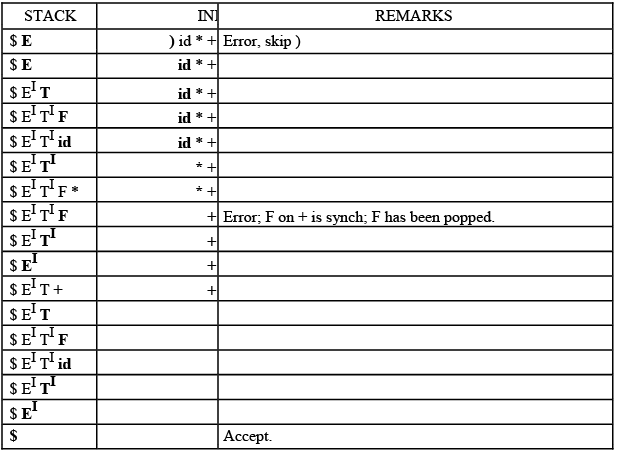Table :  Parsing and error recovery moves made by predictive parser

The document Top Down Parsing Notes | Study Compiler Design - Computer Science Engineering (CSE) is a part of the Computer Science Engineering (CSE) Course Compiler Design.
All you need of Computer Science Engineering (CSE) at this link: Computer Science Engineering (CSE)Use Code STAYHOME200 and get INR 200 additional OFF Use Coupon Code

Top Courses for Computer Science Engineering (CSE)Compiler Design

16 videos|44 docs|29 tests

Top Courses for Computer Science Engineering (CSE)Track your progress, build streaks, highlight & save important lessons and more!

,

,

,

,

,

,

,

,

,

,

,

,

,

,

,

,

,

,

,

,

,

;